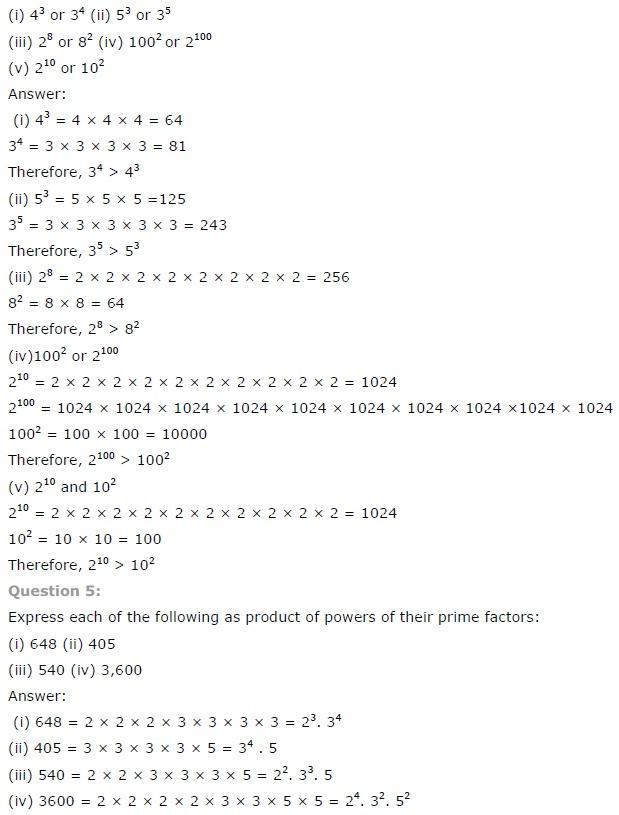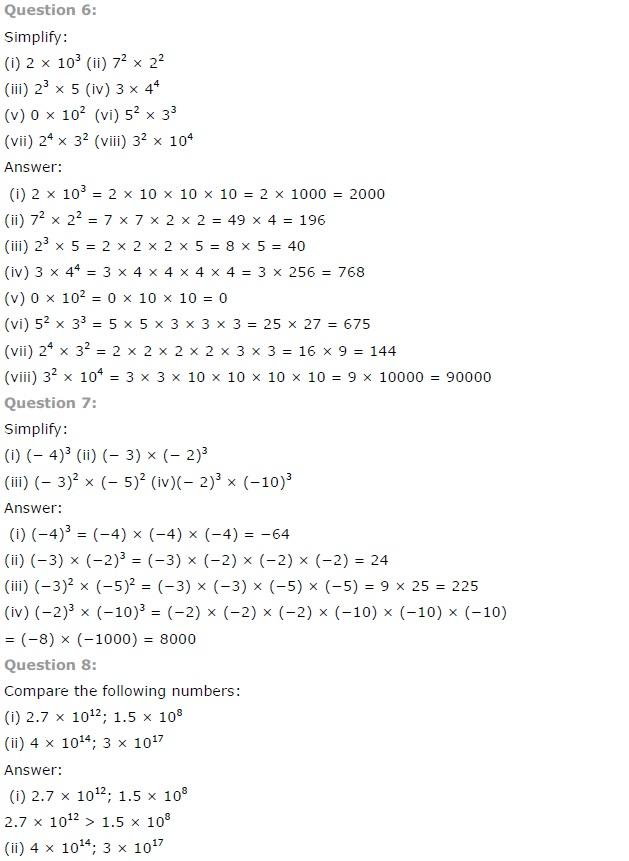# NCERT Solutions class 7 Maths Chapter-13 Exercise 13.1

## NCERT Solutions class 7 Maths Chapter-13 Exponents and Powers

### Exponents and Powers Exercise-13.1

NCERT Solutions Class-7 Maths chapter-13 Exponents and Powers Exercise-13.1 is prepared by academic team of pw all the questions of NCERT text book are solved step by step with proper and detail solutions explaining each and every questions . For More and additional questions of CBSE class 7 maths you can go to class 7 maths sections. NCERT class 7 Maths Solutions is the best way to enhanced your mathematics skill. And pw practice worksheet & question bank will help you a lot .

### Solutions of Chapter Exponents and Powers Exercise-13.1

Question 1:

Find the value of:

(i) 26

(ii) 93

(iii) 112

(iv) 54

(i)In the given question,

We have to find the value of 26

We have,

26 = 2 × 2 × 2 × 2 × 2 × 2= 64

(ii) In the given question,

We have to find the value of 93

We have,

93 = 9 × 9 × 9 = 729

(iii) In the given question,

We have to find the value of 112

We have,

112 = 11 × 11 = 121

(iv) In the given question,

We have to find the value of 54

We have,

54 = 5 × 5 × 5 × 5= 625

Question 2:

Express the following in exponential form:

(i) 6 × 6 × 6 × 6

(ii) t × t

(iii) b × b × b × b

(iv) 5 × 5 × 7 × 7 × 7

(v) 2 × 2 × a × a

(vi) a × a × a × c × c × c × d

(i) In the given question,

We have to express the given expression into exponential form

We have,

6 × 6 × 6 × 6 = 64

(ii) In the given question,

We have to express the given expression into exponential form

We have,

t × t = t2

(iii) In the given question,

We have to express the given expression into exponential form

We have,

b × b × b × b = b4

(iv) In the given question,

We have to express the given expression into exponential form

We have,

5 × 5 × 7 × 7 × 7 = 52 × 73

(v) In the given question,

We have to express the given expression into exponential form

We have,

2 × 2 × a × a = 22 × a2

(vi) In the given question,

We have to express the given expression into exponential form

We have,

a × a × a × c × c × c × c × d = a3 × c4 × d

Question 3:

Express each of the following numbers using the exponential notation:

(i) 512

(ii) 343

(iii) 729

(iv) 3125

(i) In the given question,

We have to express the given numbers into exponential notation

We have,

512 = 2 × 2 × 2 × 2 × 2 × 2 × 2 × 2 × 2 = 29

(ii) In the given question,

We have to express the given numbers into exponential notation

We have,

343 = 7 × 7 × 7 = 73

(iii) In the given question,

We have to express the given numbers into exponential notation

We have,

729 = 3 × 3 × 3 × 3 × 3 × 3 = 36

(iv) In the given question,

We have to express the given numbers into exponential notation

We have,

3125 = 5 × 5 × 5 × 5 × 5 = 55

Question 4:

Identify the greater number, wherever possible, in each of the following.NCERT CLASS 7 MATHEMATICS SOLUTIONS